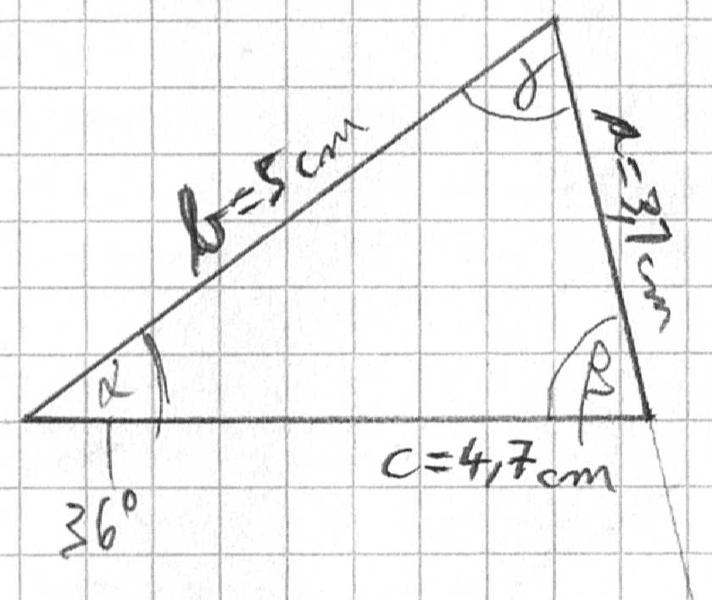# Law of sines.... I don't get it

• Aidyan

## Homework Statement

Given is a triangle with sides a=3.1cm, b=5cm, c=4.7cm and opposite angle to side a, α=36°. I must find out for angles β and γ using the law of sines.

Law of sines.

## The Attempt at a Solution

[/B]
I first tried:
sin(γ)}/c=sin(α)/a ⇒ γ≈63.02°
β=180-α-γ=80.98°

However, one could also calculate:
sin(β)}/b=sin(α)/a ⇒ β≈71.45°
γ=180°-α-β=72.55°

Two approaches, that I believed to be equivalent, but which furnish different results. So, I obviously make something wrong. But ... I don't get it... what is it?

I think you have applied the law of sines correctly. The problem is that the triangle you have given is impossible. Try applying the law of cosines to the values you have given.

Last edited by a moderator:
I draw the triangle on a piece of paper. What is wrong with this drawing?#### Attachments

Try measuring side a. If you look at it, side is is more like 3.0-3.1, not 3.7. This is also what the law of cosines would tell you.

Edit: I see now that I misread your 3.1 as a 3.7. If you accept your values of 36 degrees, 5.0 and 4.7, then a must be 3.011. If you use this value, it all works out.

Last edited:
Note that once you have specified b, c, and alpha, this fixes the shape of the triangle. The other side and the other two angles are then determined. You can't make them whatever you want.

Ahhh... now I see that.Of course, I first draw the triangle, then took the measures of all three sides and checked... but obviously that couldn't work that way. Thanks so much!